# Consider the "Deboo" integrator for unipolar noninverting designs

## Maxim MAX4250 MAX4624

The design of a standard inverting integrator is simple when bipolar supplies are available, but it's cumbersome with a unipolar supply. To allow adequate headroom for the output, the circuit must be biased away from ground, often at half the supply voltage. Because neither the input nor output is referenced to ground, the design becomes awkward and often impractical.Figure 1. The “Deboo” integrator consists of a Howland current source and a capacitive load.

One solution is a Howland current source with a capacitive load, also known as a "Deboo" integrator (Fig. 1). Powered by bipolar or unipolar supplies, this noninverting circuit has both a ground-referenced input and output. An intuitive understanding can be gained by considering the circuit in three parts: the input resistor, R; the integrating capacitor, C; and the right side of the circuit, which is equivalent to a negative resistor connected to ground.

The right side of the circuit (Fig. 2a) is easily simplified. Its defining equations are: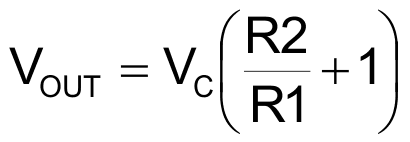and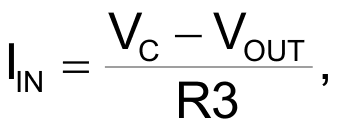resulting in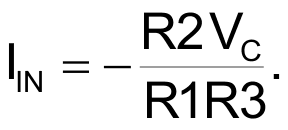Note that the input current equals the input voltage divided by a negative resistor. The negative resistor merely changes the direction of the input current. The corresponding equivalent circuit is inferred by inspection (Fig. 2b).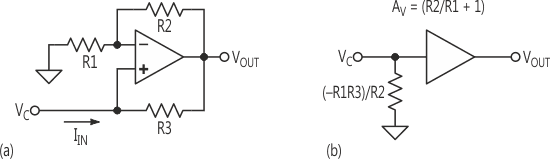Figure 2. In the circuit in Figure 1, the right-hand side (a) can be simplified to an equivalent circuit (b).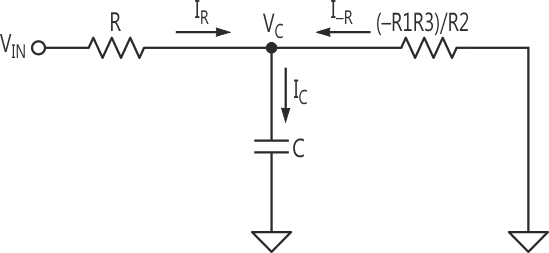Figure 3. This circuit results from a further simplification of Figure 2b.

After simplification, the circuit (Fig. 3) reveals a "T" configuration, in which VIN drives the capacitor through a positive resistor, R, and ground drives the capacitor through a negative resistor, –R1R3/R2. The total current into the capacitor is: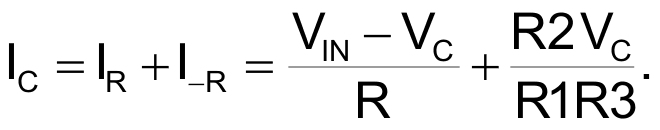If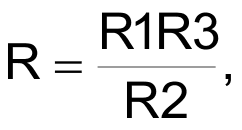then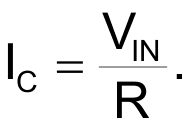This important result shows that if R = (R1R3)/R2, then current into the capacitor depends only on the input voltage VIN and R, not on the capacitor voltage. In other words, the capacitor is driven by a current source whose value is VIN/R. If VIN is a function of time, the voltage across the capacitor is: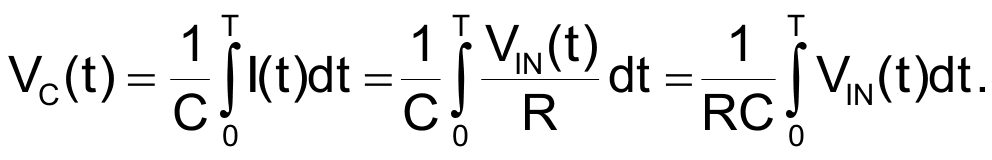VC is then amplified by (1 + R2/R1), so VOUT is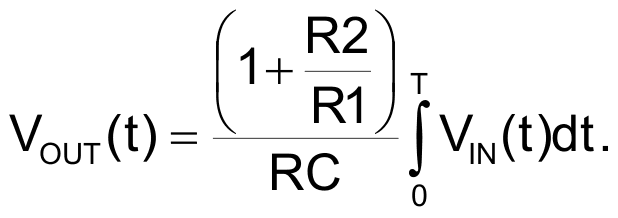The circuit shown in Figure 4 is a practical Deboo integrator with two inputs and a reset. The input R is simply the parallel combination of the two 20-kΩ resistors. The circuit can sum any number of inputs as long as the parallel combination of the input resistors equals the required R, which in turn equals R1R3/R2.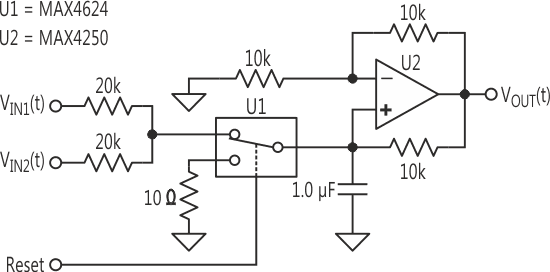Figure 4. This practical implementation of a Deboo integrator has two analog inputs and a reset input.

A single-pole/double-throw analog switch was chosen to perform the reset function. This configuration (versus a single switch across the capacitor) eliminates any offset error due to voltage division between the input R and on-resistance in the switch. To prevent the switch from going into its short-circuit-protection mode during reset (which prolongs the reset), the voltage across the MAX4624 is limited to less than 0.6 V by adding 10 Ω in series. (For capacitors of 0.1 µF or less, this voltage-limiting resistor is unnecessary).

Many parameters should be considered when designing an integrator. Fast integrators require wideband op amps with low bias-current. Very slow integrators require closely matched resistors, op amps with extremely low bias-current, capacitors with low leakage-current, and a physically clean board layout. Polypropylene, polystyrene, or teflon are the dielectrics of choice for capacitors with low leakage and low dielectric absorption.

To implement a single-input integrator, the four resistors can have the same value. Quad equal-value resistor packs with ratio tolerances down to ±0.01% are available from Caddock Electronics. Less demanding requirements can allow the use of four discrete ±1% resistors. The reset switch should be chosen carefully, since its off-leakage is integrated by the capacitor. The switch on-resistance must be low enough to allow the capacitor to be reset as quickly as necessary. Finally, the charge injected by the switch during turn-off must be sufficiently low to avoid an objectionably large offset on the capacitor.

The Deboo integrator is an easily implemented and very useful circuit, particularly for single-supply applications.

## Materials on the topic

electronicdesign.com